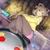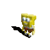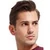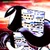24,835 Pages

## What is a calculation?

A calculation (referred to as calc for short) is a method of determining a statistic (referred to as stat for short) of a character (usually Attack Potency or Speed) in cases where it cannot be directly determined. It makes use of scientific formulae and mathematical equations to calculate any particular stat of the character.

## Benefits of a calculation

Since scientific formulae and mathematical equations are universally standard and objective (i.e. not subject to either opinions or individual perspectives), they are accepted as true and not subject to debate by either supporters or opponents of the fictional series. In simplified terms, a calc is the best and most objective method to either prove or disprove a statement, or end a debate as it were.

## Drawbacks of a calculation

Of course, not everything is that simple. Even calculations have their own drawbacks. For one, calculations generally involve pixel scaling (more of that later on) which is hard to measure and utilize. Calculations also make presumptions beforehand which are subject to much debate (and are hence not argument-proof). Last of all, calculations are tough. To not only calculate a stat, but to also convey the procedure step by step is tricky and often leaves people confused if done incorrectly.

## Frequently Used Measuring Techniques

### Pixel Scaling

As we have discussed in the calculation methods page, we will most likely need to determine volumes of various objects in order to determine destructive capacity, and as we've also discussed, volume is composed of three perpendicular dimensions, and in order to find the lengths of those dimensions, we will use Pixel Scaling.

Pixel Scaling works much like the scales we have on maps, in fact it's where the name of the term comes from. In maps you the scale that states how many Kilometers/meters one Centimeter on the map represents in the real world, in Pixel Scaling we try to find how many kilometers/meters/centimeters one pixel represents on a certain scan, and we will find said ratio by using measuring stick.

A measuring stick (not to be confused with the real life measuring sticks and rulers) is basically something that we already know the size of, and can measure how many pixels in the scan it takes to cover said size, for example; if a human whose height is 172 centimeters would be portrayed as 172 pixels high in a certain, the scale would be one centimeter per pixel in said scan.

However, there are some flaws in Pixel Scaling, such as the fact that we measure feats in 3 dimensions, while scans of comics and manga are 2 dimensional, that is why we need to make sure that every object we scale must be in close proximity to our measuring stick.

### Angular Size

A less reliable, but often useful technique is angular sizing or angsizing for short. By assuming a typical human field of view it allows one to estimate the size of an object if one knows the distance from the point of view or estimate the distance to the point of view if one knows the size of the object.

To do this, you need to use several steps:

1. Find or estimate the size of the object or the distance to the object.
2. Measure the pixel size of the object and the pixel height of the screen (not the black area, merely the area where objects are).
3. Plug into this equation to get degrees: 2atan(tan(70deg/2)*[object size in pixels/panel height in pixels]). Make sure your calculator is calculating the atan and tan in degrees, not radians.
4. Navigate to the angsize calculator and pick what you wish to solve for.
5. Plug in your degrees and the size/distance from step 1 and your result will be displayed in whatever units that was in.

This method gives a solution to the problems that sometimes occur in pixel scaling, but it is important to remember that if both a direct pixel scaling measurement for a certain object in one scan and an angular size measurement for said object from another scan are taken, the pixel scaling takes precedent and should be treated as more accurate.

Alternatively to using the calculator one can also directly plug the values into these formulas to solve everything in one step:

• Distance from point of view to object = object size * panel height in pixels/[object height in pixels*2*tan(70deg/2)]
• Object size = 2*tan(70deg/2) * distance from point of view to object * object height in pixels / panel height in pixels

In both formulas the unit of object size is the same as the unit of the distance from the point of view to the object. In these formulas as well make sure your calculator is calculating the tan in degrees, not radians.

You can also use this angsizing method to find the distance between two objects shown in the panel, if you happen to know or can estimate the size of the two objects proper. Simply:

1. Angsize the distance between the point of view and each of the objects.
2. Find the positive difference between the two values. This is the distance between the two objects in the panel.

However, note that this is only possible if the objects are not very close to each other and form no significant angle between each other from the camera angle. If that is the case, geometry will be required to solve for the true distance. Ask the calc group for assistance in this scenario.

## Starting a calculation

Start a calculation by first obtaining three things:

a) Presumptions: Every calculation needs a certain set of presumptions to justify the subsequent calc. List your presumptions clearly and state your reasons for the presumption.

b) Scans: If your calculation makes use of pictures (such as manga panels), it is imperative that you present it to the community for first-hand viewing.

c) Scientific guides/articles: If your calculation makes use of any scientific formula which is obscure, present a link to the formula in question.

Once all three are obtained, present your calculation.

## The calculation

Display every step of your calculation (equations and such), along with a sentence in order to explain the workings of your calc. Also try to explain the science behind your calc as you go along. Make sure to highlight important figures and equations which are to be utilized repeatedly. Also, highlight all end results for fast viewing.

## After the calculation

Once your calculation is done, invite community members to inspect your calc, who will do so. The community may point out any errors in the calc or in the presumptions before it. Try and explain your point if there is a misunderstanding. In case of any errors, correct them. In case of a discrepancy, members can ask Admins to present their opinions on the matter.

## Conclusion

At the end of a calculation, if it accepted by the community, link the relevant pages to the calculation in question. If otherwise, try to find out the reasons for discarding your calc and next time, make a calc keeping them in mind.

## Start a Discussion Discussions about Calculation Guide

•#### Calculation Evaluations - 18

57 messages
•'''Priority:''' 1 (Scales to all characters) '''Verse:''' Azure Striker Gunvo...
•Nullflowerblush wrote:* '''Priority:''' 2 (The first few scale to both...
•#### References for Common Feats Requests and Evaluations Thread 3

26 messages
•That's around 32,687,500 Joules. Around the middle of Small Building level.
•Still waiting for the wooden chair fragmentation feat. Specifically, something like this chair:...
Community content is available under CC-BY-SA unless otherwise noted.